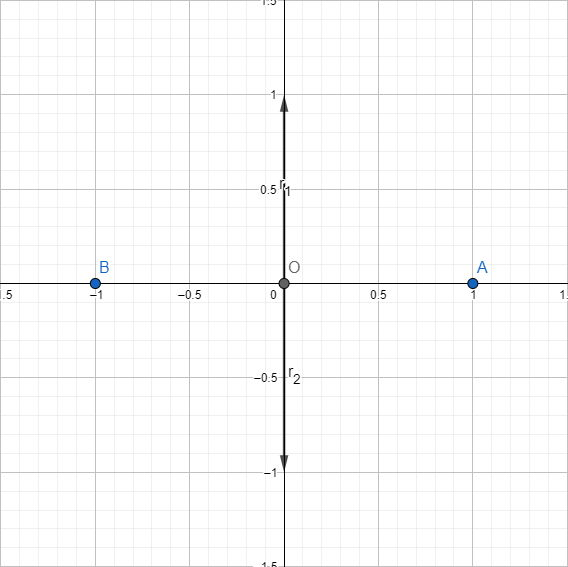시간 제한메모리 제한제출정답맞힌 사람정답 비율
2 초 512 MB214325.000%

## 문제

Planet Oz is located in the origin of two-dimensional euclidean space. Elphaba wants to show everyone that her powers lie far beyond the laws of physics. To do this, she wants to take off from Oz and fly away along some straight line to infinity and beyond.

The task is complicated by the fact that Oz has n satellites revolving around it. For each satellite, its polar coordinates (ρi; φi) and mass mi are known. Here, the planet and satellites are considered points, ρi is the radial distance from Oz to the i-th satellite, and φi is the polar angle between the satellite and some fixed reference direction measured in arc seconds.

Assume that Elphaba is located in the point denoted by vector $$\vec{r}$$ and has mass m, while i-th satellite is located in the point denoted by vector $$\vec{r}_i$$. The satellite attracts Elphaba with the force directed from Elphaba to the satellite. This force is proportional to Elphaba’s mass m and satellite’s mass mi, and inversely proportional to the squared distance between them, |$$\vec{r}$$ − $$\vec{r}_i$$|2. Thus, exact value of force $$\vec{F}_i$$ applied to Elphaba by i-th satellite is as follows:

$\vec{F}_i = - \frac{Gmm_i(\vec{r} - \vec{r}_i)}{|\vec{r} - \vec{r}_i|^3}$

Here, G is the universal gravitational constant, the value of which does not concern us in this problem.

Forces applied from different satellites sum up directly. Therefore, the overall force $$\vec{F}$$ applied to Elphaba by all satellites is equal to $$\vec{F} = \vec{F}_1 + \cdots + \vec{F}_n$$.

Elphaba wants to choose the direction of her flight in such a way that it doesn’t pass through any of the satellites, and its direction is not affected by satellites at any moment of time. In other words, the force $$\vec{F}$$ must be collinear to  $$\vec{r}$$ for all points $$\vec{r}$$ on Elphaba’s trajectory.

## 입력

The first line of input contains a single integer n (2 ≤ n ≤ 2 · 105), the number of satellites.

Each of the following n lines contains three integers ρi, φi, and mi (1 ≤ ρi ≤ 1018, 0 ≤ φi < 129 600, 1 ≤ mi ≤ 109) representing polar coordinates and mass of the i-th satellite.

It is guaranteed that no two satellites have the same polar angle φ.

## 출력

Output a sorted list of real numbers α (0 ≤ α < 129 600) denoting polar angles in arc seconds of all possible flight directions. Your answer would be considered correct if absolute or relative error of every number in the list is at most 10−6. It is guaranteed that the answer always contains at most 2 · 105 directions.

## 예제 입력 1

2
1 0 1
1 64800 1


## 예제 출력 1

2
32400.0000000
97200.0000000


## 예제 입력 2

3
1 0 1
1 1 1
1 2 1


## 예제 출력 2

1
64801.0000000


## 힌트

One arc second (denoted as 1′′) is equal to one sixtieth of one arc minute (denoted as 1′), which in turn is equal to one sixtieth of one arc degree (denoted as 1). Therefore, 60′′ = 1′, 60′ = 1, and 129 600′′ = 360, which means that 129 600 arc seconds constitute the full arc.In the first example, the first satellite is located at point A(1; 0), and the second one is located at point B(−1; 0). The only possible flight directions on the picture are denoted by $$\vec{r}_1$$ and $$\vec{r}_2$$ having polar angles 90 = 32 400′′ and 270 = 97 200′′ correspondingly.